Force FluctuationsAll forces discussed so far are averages of a fluctuating quantity.As a simple consequences of such fluctuations consider the wanderings of

a classical particle of mass m in a quantum bath.There is no net force on the particle, but it disperses due to force fluctuations: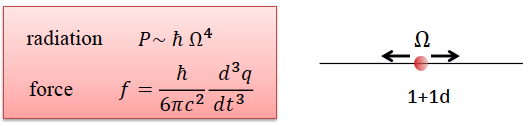Langevin equation for an otherwise free particle,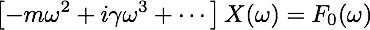In the commonly used Caldeira-Legget formalism, the force is linearly coupled to the bath,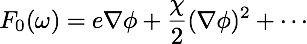However, a linear coupling is forbiddent for a neutral, but polarizable, particle.With linear coupling, a free field theory implies a Gaussian distribution for φ,

hence the force and the displacement are also Gaussian distributed, entirely described by the variance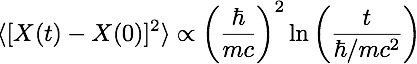For a neutral particle (no linear coupling), the quadratic force is not Gaussian distributed; leading to cumulants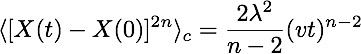consistent with a power-law probability distribution, reminiscent of Levy flights:Potential realizations for solitons in Bose-Einstein condensate.